# 学习Tkinter图形界面编程，编一个简单的计算器（一）——界面部分

in #cn2 years ago

Tkinter是python自带的图形界面库，虽然功能简单，但强在简单易学，开箱即用，安装python时已经自带了，不需要额外安装。下面我们就通过编一个简单的计算器来学习Tkinter图形界面编程的基本知识。

## 1.Tkinter的编程哲学：

Tkinter里面一切都是窗体+小部件，小部件种类很多，有标签、按钮、输入栏、菜单、画布。。。而Tkinter的编程哲学简单概括就是：

• 1、要有窗体
• 2、定义一个部件
• 3、把部件放到窗体上

## 2、Tkinter编程实例：

### （1）要有窗体

``````from Tkinter import *

root=Tk()

root.mainloop()

``````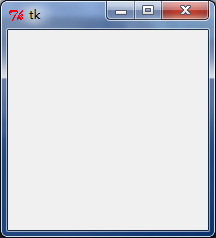### （2）定义一个部件：

``````from Tkinter import *

root=Tk()

#######################
e=Entry(root,width=50)
#######################
root.mainloop()
``````

### （3）把部件放到窗体上：

Tkinter中把部件放到窗体上有几种方式，常见的是两种：打包（pack）、网格（grid）。打包非常简单，就是按先后顺序堆放。网格的控制能力强一些，在后面的计算器部分再讲。这里我们直接把输入框“打包”到窗体上，也是只要一行代码就行：

``````from Tkinter import *

root=Tk()

#####################
e=Entry(root,width=50)

e.pack()
#####################
root.mainloop()

``````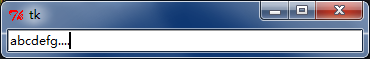## 3、计算器的界面编程

### （1）网格（grid）布局方式：

``````e.grid(row=0,column=0)
``````

### （2）添加数字按钮：

``````btn7=Button(root,text='7',width=16,command=add)

btn7.grid(row=1,column=0)
``````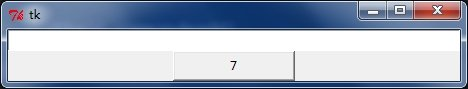``````#-*-coding:utf-8 -*-
from Tkinter import *

return
root=Tk()

e=Entry(root,width=64)
e.grid(row=0,column=0)

#设置按键

btn7.grid(row=1,column=0)
btn8.grid(row=1,column=1)
btn9.grid(row=1,column=2)
btn4.grid(row=2,column=0)
btn5.grid(row=2,column=1)
btn6.grid(row=2,column=2)
btn1.grid(row=3,column=0)
btn2.grid(row=3,column=1)
btn3.grid(row=3,column=2)
root.mainloop()
``````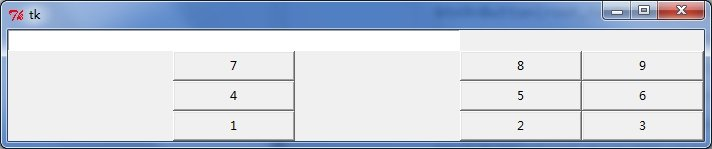``````e.grid(row=0,column=0,columnspan=4)
``````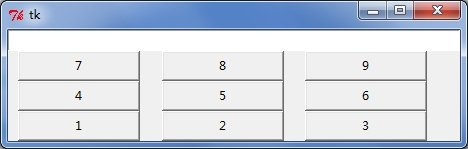``````#-*-coding:utf-8 -*-
from Tkinter import *

return
root=Tk()
root.title('计算器')

e=Entry(root,width=64)
e.grid(row=0,column=0,columnspan=4)

#设置按键

btn7.grid(row=1,column=0)
btn8.grid(row=1,column=1)
btn9.grid(row=1,column=2)
btn4.grid(row=2,column=0)
btn5.grid(row=2,column=1)
btn6.grid(row=2,column=2)
btn1.grid(row=3,column=0)
btn2.grid(row=3,column=1)
btn3.grid(row=3,column=2)
btn_sub.grid(row=2,column=3)
btn_product.grid(row=3,column=3)
btn_divide.grid(row=4,column=3)
btn_equal.grid(row=4,column=2)
btn_clear.grid(row=4,column=0,columnspan=2)
root.mainloop()
``````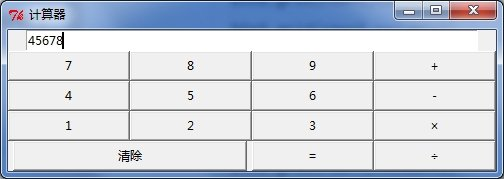## 参考资料：

Sort:

!thumbup

@justyy 是CN区的见证人，请支持他，给他投票，或者设置justyy为见证人代理。感谢！@justyy的主要贡献：https://steemyy.com

Congratulations! This post has been selected by @justyy as today's (2019-12-01) 【Good Posts】, Steem On! Reply to this message in 24 hours to get rewards. Upvote this comment to support the @dailychina and increase your future rewards! ^_^

If you believe what I am doing, please consider a spare vote voting me here, thank you very much indeed.

@justyy - the author of https://SteemYY.com and I have been a Steem Witness for more than a year now.

JCAR 11월 구독보팅입니다.
어느새 11월 마지막주가 되었네요. 즐거운 한 주 되시기바랍니다.This post has been voted on by the SteemSTEM curation team and voting trail. It is elligible for support from @curie and @minnowbooster.

If you appreciate the work we are doing, then consider supporting our witness @stem.witness. Additional witness support to the curie witness would be appreciated as well.